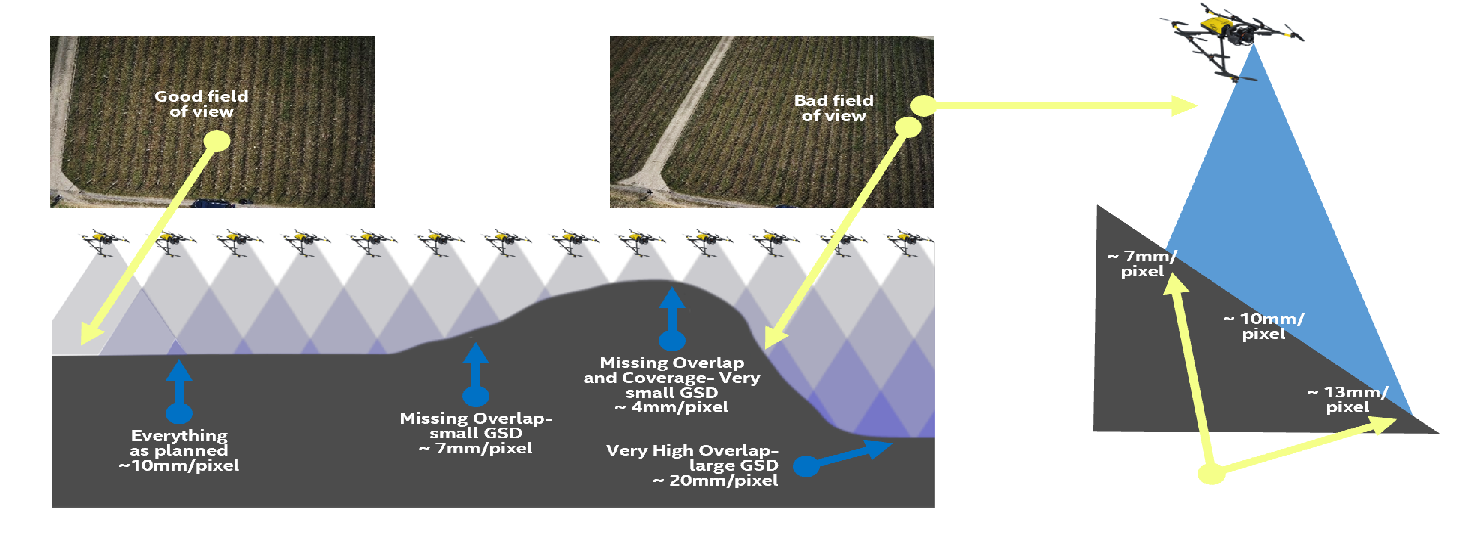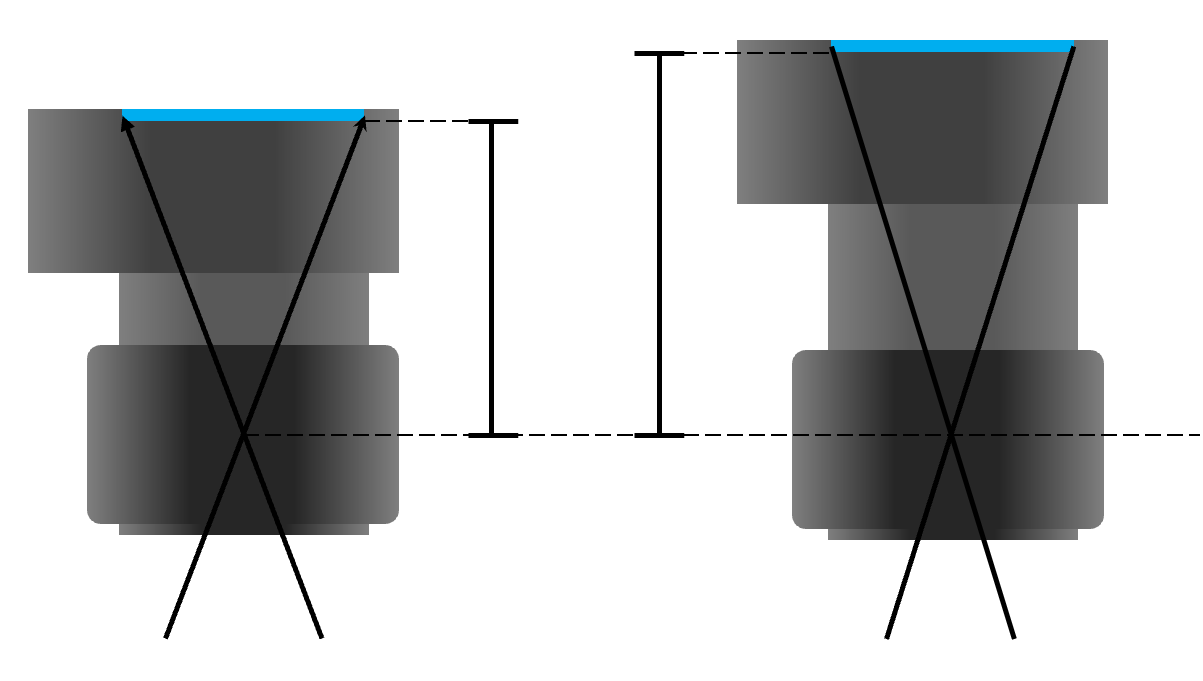# Compute Flight Height for Ground Sampling Distance

เอกสาร

การติดตั้งและตั้งค่า

000028551

22/07/2019

Ground Sampling Distance (GSD): the real world space that each pixel represents• A: Altitude (meters)
• GSD: Ground Sampling Distance (cm/pixel)
• f: Focal Length (mm)
• ps: Pixel Size (microns, nanometers, etc)The flight height H that is needed to obtain a given GSD can be computed and depends on the camera focal length, the camera sensor width [mm], and the image width [pixels].

Sw = real sensor width [mm]

FR = real focal length [mm]

H = flight height [m]

Dw = distance covered on the ground by one image in the width direction (footprint width) [m]Some lens manufacturers give the focal length (F35) in the 35 mm equivalent. You shouldn't use the 35 mm equivalent, but the real focal length in Pix4Dmapper. To find the real focal length, some computations are needed. In the case of a 4:3 ratio, the formula for the real focal length FR is given by:

FR [mm] = (F35 * SW) / 34.6 (1)

This means:

F35 = focal length that corresponds to the 35 mm equivalent

FR = real focal length

Sw = the real sensor width

Using the fact that

H / FR = DW / SW,

the flight height H is given by:

H = (DW * FR) / SW . (2)

The distance covered on the ground by one image in the width direction (footprint width) is given:

DW = (imW * GSD) / 100. (3)

where

DW = distance covered on the ground by one image [m] in the width direction (footprint width)

imW = image width [pixel]

GSD = desired GSD [cm/pixel]

Combining equation (2) and (3), the flight height is given by:

H [m] = (imW * GSD * FR) / (SW * 100) (4)

 Note The result is given in [m], considering that the GSD is in [cm/pixel].

Example:

Computation of the flight height to get a GSD of 5 [cm/pixel] using a camera with a real focal length of 5 [mm] and a real sensor width of 6.17 [mm]. Assuming that the image width is 4000 [pixels] and using the equation (4), the flight height should be 162 [m].

H = (imW * GSD* FR ) / (Sw * 100) = (4000 * 5 * 5) / (6.17 * 100) = 162.07 [m]